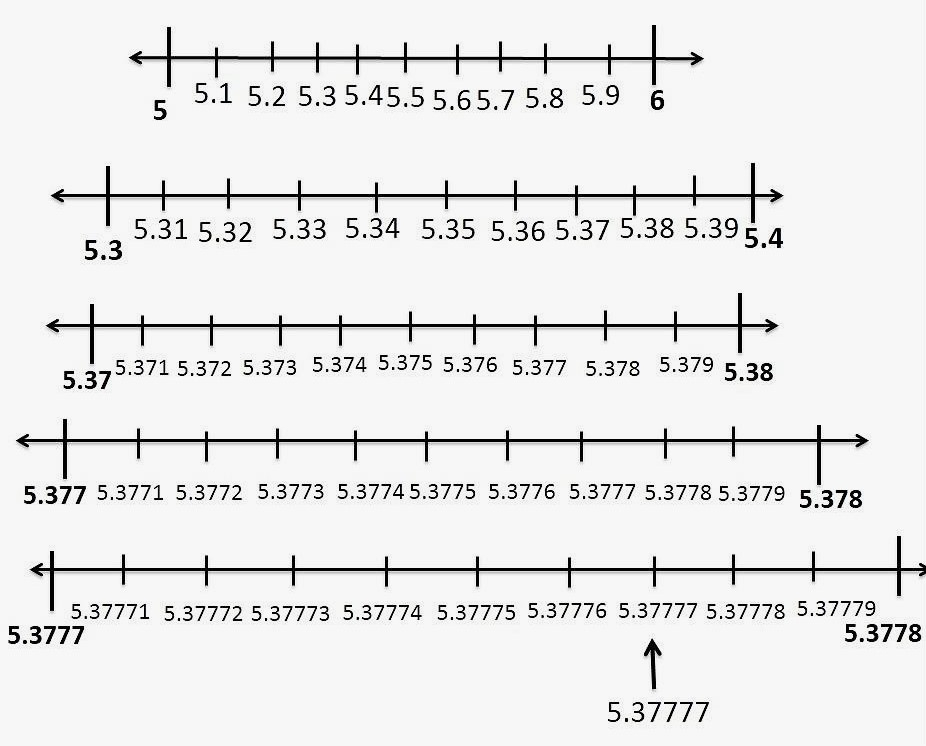# Visualise the representation of $5.3\overline{7}$ on the number line upto 5 decimal places, that is upto 5.37777.

Given:

Given number is $5.3\overline{7}$.
To do:

We have to visualise the representation of $5.3\overline{7}$ on the number line upto 5 decimal places, that is upto 5.37777.
Solution:

$5.3\overline{7}$ lies between 5 and 6.

So, we divide the number line into 10 equal parts and mark each point of division. The first mark on the right of 5 will be $5.1$ followed by $5.2$ and so on.

The point left of 6 will be $5.9$.

The magnified view of the above will show that $5.37777$ lies between 5.3 and 5.4.

Divide the line segment between 5.3 and 5.4 into 10 equal parts.

The first part will be 5.31 followed by 5.32  and so on.

Following the above process five times, we get,

5.37777 will be 7th mark in this subdivision as shown in the figure.Updated on: 10-Oct-2022

24 Views